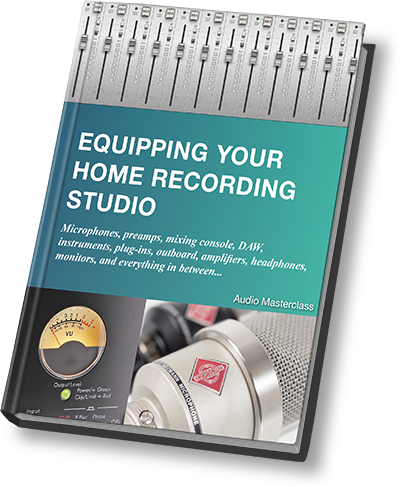# Additive Synthesis - how does that work?

## The first practical method of electronic sound synthesis was the subtractive synthesizer. Before that however, there was additive synthesis - the problem was that it just didn't work...In the days before the legendary work of Robert Moog, inventor of the Moog synthesizer, the quest was on for a versatile electronic means of sound generation. Although there were already electric and electronic keyboard instruments, no-one had yet devised a true synthesizer capable of a massively wide range of sound textures.

One idea was additive synthesis. Additive synthesis exploits the fact that any complex sound can be analyzed into its component sine waves (a sine wave is the simplest sound, consisting of only one frequency). Mathematically the reverse would also be true - any sound can be constructed out of sine waves of the right frequencies, levels and phase relationships (timing).

The problem back in the early days was that analog electronics were not up to the job. It was impossible to get sufficient accuracy to make additive synthesis work. However, digital electronics can perform additive synthesis just fine.

So a digital additive synthesizer will consist of many sine wave oscillators (obviously created by calculation rather than components, but the concept holds true). The frequencies, levels and phase relationships are precisely controlled to construct the desired sound. For example a square wave can be made from a fundamental frequency, the third harmonic which is 3x that frequency, the fifth harmonic which is 5x that frequency, and so on with all the odd whole number multiples of the fundamental.

## FREE EBOOK - Equipping Your Home Recording StudioPlainly, a lot of oscillators will be necessary to cover the whole audio bandwidth, and this leads to the requirement for a lot of envelope generators as well. Where a subtractive synthesizer (commonly known as an 'analog synthesizer') can get by quite well with just two envelope generators, an additive synthesizer requires one envelope generator per sine wave oscillator so that the level of each of the harmonics can be controlled independently.

In fact, done properly, each oscillator should have the following:

• amplifier
• pitch envelope generator
• pitch LFO
• volume envelope generator
• volume LFO

A pure additive synthesizer does not require a filter of any kind. Sound creative is totally additive - nothing needs to be filtered away.

Additive synthesis works very well - an example of a practical additive synthesizer is the Kawai K5. However one problem is in constructing highly complex noise-like sounds. In theory this can be done via additive techniques, but it is easier just to have a noise generator, then filter the noise as in subtractive synthesis.